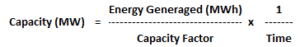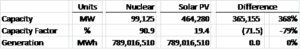# Capacity Factor – Part 2

In our previous article we looked at Capacity Factor and how it differs between nuclear generation and solar PV (photovoltaic).   We concluded that in order to generate the same amount of electricity as 1/3 of the capacity of the US nuclear generation fleet (33,042 MW), 154,760 MW of solar PV capacity would be required.  This is a result of the substantially different Capacity Factors of nuclear (90.9%) and solar PV (19.4%) and is summarized in the table below.A reader asked how much solar PV capacity would be needed in order for solar PV to generate as much electricity as the entire US nuclear generation fleet.  As noted in the previous article, the current US nuclear generation fleet consists of 100 operating units with a combined capacity of 99,125 MW which, during 2013, produced 789,016,510 MWh of electricity.

In order to calculate the amount of solar PV capacity needed, we can rearrange the Capacity Factor formula we used last time as follows:Solving for the solar PV capacity needed to supply the same amount of electricity as the US nuclear generation fleet, we arrive at the following:

Capacity (MW) = 789,016,510 MWh / (19.4% x 8,760 hours/year)

Capacity (MW) = 464,280

In summary, 464,280 MW of solar PV capacity would be needed in order for solar PV to generate as much electricity as the entire US nuclear generation fleet.  This is 365,155 MW more than the existing 99,125 MW of installed nuclear capacity and is summarized in the table below:As noted in our previous article, solar PV, like other sources of electricity generation (nuclear, wind, coal, natural gas, geothermal, biomass, etc.) comes with a set of tradeoffs.  Each source has its own strengths and weaknesses.  The focus here is simply on Capacity Factor.

The Avalon Advantage – Visit our website at www.avalonenergy.us, call us at 888-484-8096, or email us at jmcdonnell@avalonenergy.us.

Notes:

Data from the US Energy Information Administration